# Points, Lines And Planes

Points, Lines and Planes are the undefined terms that provide the starting place for geometry or plane geometry. One of the easiest sections in CAT quantitative aptitude is points, lines and planes. These topics are grouped together since they deal with the portion of Quantitative Aptitude that can be visualized. The definition and various properties of points, lines and planes are discussed below.

### Points

A point is thought of as a “dot” on a piece of paper. A point has no length, width, or height. It just specifies an exact location. It is zero-dimensional. The following is a diagram of points A, B, and M.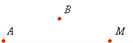### Lines

A line is one-dimensional. That is, a line has length, but no width or height. A line extends forever in both directions. A line is uniquely determined by two points.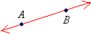The line passing through the points A and B is denoted by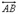### Line Segment

A line segment connects two endpoints. A line segment with two endpoints A and B is denoted by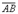.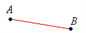A line segment can also be drawn as part of a line.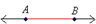### Mid-Point

The midpoint of a segment divides the segment into two segments of equal length. The diagram below shows the midpoint M of the line segment. Since M is the midpoint, we know that the lengths AM = MB.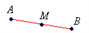### Rays

A ray starts from one endpoint and extends forever in one direction.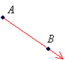A ray starting from point A and passing through B is denoted by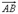. Planes are two-dimensional. A plane has length and width, but no height, and extends infinitely on all sides. Planes are thought of as flat surfaces, like a tabletop. A plane is made up of an infinite amount of lines. Two-dimensional figures are called plane figures.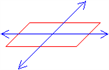### Space

Space is the set of all points in the three dimensions – length, width, and height. It is made up of an infinite number of planes. Figures in space are called solids.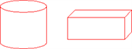Stay tuned with BYJU’S to get the latest updates on CAT 2021. Learn various other important topics of CAT quantitative aptitude section at BYJU’S.